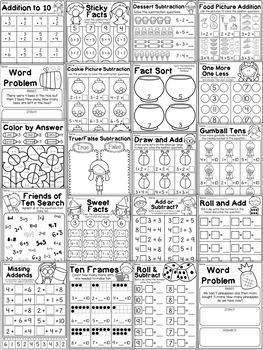# Kindergarten Addition and Subtraction Worksheets (up to 10)PreK - 1st
Subjects
Resource Type
Formats Included
• PDF
Pages
36 pages

#### Also included in

1. This bundle is packed with so many fun-filled math worksheets for kindergarten students. It is perfect for whole-class activities, math stations, fast finisher activities, homework and review. The worksheets cover addition and subtraction to 10, place value, 2D & 3D shapes and numbers to 20. A t
\$10.00
\$13.75
Save \$3.75

### Description

This packet is jammed full of addition and subtraction worksheets to help your students master the basic facts. It includes 36 engaging worksheets which cover both addition and subtraction facts up to 10. Students will be practicing picture addition/subtraction, using number lines, finding missing addends, making ten and so much more! It is perfect for whole-class activities, math stations, fast finisher activities and review.

Save 30% with the bundle!

Kindergarten Math Worksheet MEGA Bundle

The worksheets include:

♥ Food Picture Addition – Use the food pictures to work out the addition questions.

♥ Picture Addition Fun - Use the pictures to work out the addition questions.

♥ Draw and Add – Draw dots on the ladybugs’ wings to work out the addition questions.

♥ Ten Frames – Make ten by drawing more dots in the ten frames.

♥ Gumball Tens – Draw more gumballs to make ten.

♥ Friends of Ten Search – Highlight all of the equations that make ten.

♥ Bright Pencils – Use the key to color the pencils.

♥ Apple Seed Bonds – Write the missing number to complete the bond.

♥ Sticky Facts – Color the facts that match the answer.

♥ Roll and Add – Roll 2 die, write the numbers, answer the equation.

♥ Color by Answer – Color the picture using the key (addition version).

♥ True or False Addition – Sort the questions into the correct column.

♥ One More, One Less – Write the number that comes before and after each number.

♥ Add or Subtract – Draw a plus or minus sign to complete the equation.

♥ Sporty Subtraction – Use the pictures to work out the subtraction questions.

♥ Dessert Subtraction – Use the pictures to work out the subtraction questions.

♥ Cookie Picture Subtraction – Cross out the cookies to work out the subtraction questions.

♥ School Subtraction – Fill in the missing numbers to solve the subtraction questions.

♥ Color by Answer – Color the picture using the key (subtraction version).

♥ True/False Subtraction – Sort the questions into the correct column.

♥ Sweet Facts – Color the facts that match the answer.

♥ Fact Sort- Sort the facts into the fishbowls according to their answer.

♥ Number Line Subtraction – Use the number line to work out the subtraction equations.

♥ Missing Numbers – Paste the missing numbers to complete the questions.

♥ Subtraction to 10 – Use the cupcakes to help you solve the subtraction questions.

♥ Bright Books – Color the books using the key.

♥ Roll and Subtract – Roll a die, write the number, answer the question.

♥ Subtraction Spinner – Spin the spinner, write the number, answer the question.

♥ 2 x Word Problems – Read, draw and answer the subtraction word problems.

You may also like:

Kindergarten Numbers to 20 Worksheets

Kindergarten 2D and 3D Shapes Worksheets

Kindergarten Place Value Worksheets

First Grade 2D and 3D Shapes Worksheets

First Grade Fractions and Partitioning Worksheets

First Grade Data and Graphing Worksheets

First Grade Numbers and Place Value Worksheets

Second Grade Place Value and Number Worksheets

♥♥♥ Earn TPT Credits when you leave feedback ♥♥♥

I welcome and appreciate any feedback, comments or suggestions.

You may also contact me on myteachingpal@outlook.com

Total Pages
36 pages
N/A
Teaching Duration
N/A
Report this Resource to TpT
Reported resources will be reviewed by our team. Report this resource to let us know if this resource violates TpT’s content guidelines.